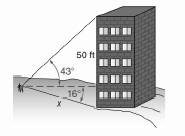Chapter 11.CR, Problem 13CRElementary Geometry For College St...

7th Edition
Alexander + 2 others
ISBN: 9781337614085

Solutions

Chapter
SectionElementary Geometry For College St...

7th Edition
Alexander + 2 others
ISBN: 9781337614085
Textbook Problem

In Review Exercises 13 to 17, use the Law of S i n e s or the Law of C o s i n e s to solve each problem. Angle measures should be found to the nearest degree; distances should be found to the nearest tenth of a unit.A building 50 ft tall is on a hillside. A surveyor at a point on the hill observes that the angle of elevation to the top of the building measures 43 ° and the angle of depression to the base of the building measures 16 ° . How far is the surveyor from the base of the building?To determine

How far is the surveyor from the base of the building.

Explanation

Procedure used:

In any triangle ABC such that

AB=c,

BC=a,

CA=b,

mA=α,

mB=β, and

mC=γ.

The Law of cosines is given by

a2=b2+c2-2bccosα

b2=c2+a2-2cacosβ

c2=a2+b2-2abcosγ

The Law of sines is given by

asinα=bsinβ=csinγ

Calculation:

Given:

Let us name the given figure as follows.

From the above figure we have two triangles having right angle at P.

Thus, in ASP we have

mASP=43°

Also, in BSP

mBSP=16°

BS=x

Then, in ASB we have

mASB=mASP+mPSB

mASB=43°+16°

mASB=59°, and

AB=50 ft.

Also BS=x.

Now, consider the triangle ASP to find the mSAP

Still sussing out bartleby?

Check out a sample textbook solution.

See a sample solution

The Solution to Your Study Problems

Bartleby provides explanations to thousands of textbook problems written by our experts, many with advanced degrees!

Get Started

Find more solutions based on key concepts